Starting is half the way. Finishing is hard.

When you google “80/20 rule”, you will find hundreds of time and project management articles explaining how to do more by doing less, e.g.,

The 80–20 rule is the principle that 20% of what you do results in 80% of your outcomes. Put another way, 80% of your outcomes result from just 20% of your inputs. Also known as the Pareto principle, the 80–20 rule is a timeless maxim that’s all about focus. Because so much of your output is determined by a relatively small amount of what you do each day, focusing on the most productive tasks will result in greater output. (Change Your Life with the 80–20 Rule)

Do you know where the 80/20 rule is actually coming from? What is the underlying theory that justifies outsourcing 80% of the work with minor consequences and focussing on the 20% that can be attributed to 80% of the impact? Where does that magic number that answers the ultimate question of life originates from?

Unfortunately, it is much harder to find references that answer this question. The motivation of that article is to provide a step-by-step derivation of the 80/20 rule — because of the author’s curiosity in the underlying math, but also to understand when to challenge the bold statement as killer argument in an arbitrary context. We will start with the math behind Pareto’s 80/20 rule and will close with a summary of the assumptions and less known implications.

The Math Behind It

The Pareto Principle is named after Vilfredo Pareto, who found that 80% of Italy’s wealth was owned by about 20% of its population at the end of the 19th century (see, e.g., wikipedia for more details). Pareto’s observation was about population and wealth not economics and business. In this section, we will formalize the statement by deriving a functional form that puts the share of the total income in relation to the proportion of the rich. This derivation is based on lectures notes from the American University.

Pareto stated that the income X is distributed not evenly across the population, but rather follows a power law distribution, i.e., a high share of the total income is owned by a relatively small proportion of the population. He suggested that the income can be modeled as a Pareto distribution:

if x≥xₘ and 0 otherwise, with the minimal income (scale) xₘ>0 and shape α>0.

Figure 1 shows the histogram of the monthly net income distribution per household in Germany 2013 as an illustrative example and the fitted probability density function (pdf) of the Pareto distribution.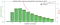Figure 1: The green bars represent the monthly income proportion of German households in 2013. The red curve is the fitted probability density function of the Pareto distribution.

The cumulative distribution function (cdf) reflects the proportion of the population with an income of at most x. The tail distribution (one minus the cdf) allows us to compute the proportion with at least an income of x:

if x≥xₘ and 1 otherwise. Figure 2 shows the empirical tail distribution of the monthly net income by household in Germany in 2013 and P(X>x; xₘ,α).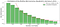Figure 2: The green bars represent the proportion of the population with more than a specific income. The red line represents the fitted tail distribution of the Pareto distribution.

This model allows us to infer the proportion of the population that has more than a specific income. In order to derive a statement relative to the total income, all incomes can be normalized by the minimal income xₘ. Thus, we can simplify the proportion of the rich in the population to

where the income x is expressed as multiples of the minimal income xₘ (see Figure 3).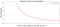Figure 3: The red curve represents the richness function n(x;α) fitted to the income sample. In this case, 40% of the population have an income of more than 2 times xₘ.

Note that the proportion n(x;α) of the population with at least x multiples of the minimal income is equivalent to the proportion n(dx;α) that has an increment of dx, i.e., the area under the curve

represents the total income in terms of multiples of the minimal incomes xₘ. Thus, the share of the total income given a specific income x, can be expressed as:

Figure 4 shows the share of total income as a function of the income multiples.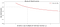Figure 4: The red curve represents the share of the total income s(x;α) fitted to the income sample. In this case, people with an income of more than 2 times xₘ account for 80% of the total income.

By comparing Figure 3 and 4, we can see that in our example 40% of the population account for 80% of the total income. Generally speaking, we can express the share of the total income s(x;α) as a function of the proportion of the rich n(x;α) by substituting the income by the inverse function n⁻¹:

The expression models how much of the total income is owned by a specific proportion of its population. Given a proportion of rich and the corresponding share of total income, we can determine α by rearranging the terms of s(n;α)

Thus, the 80/20 rule corresponds to α≈1.16. Figure 5 shows the function s(n;α=1.16). The share of total income for a larger proportion of the population is quickly decreasing (given by the derivative s’(n;α=1.16)); while 0.7% of the population already has a 50% share, a proportion of 50% accounts only for 91% of the total income.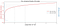Figure 5: The red line shows the function s(n;α=1.16) modeling the share of total income as a function of the proportion of the rich. The blue line is the derivative of s describing the decrease of the share of total income for a larger proportion of the population.

Let’s summarize the modeling assumptions:

• The Observations Follow a Pareto Distribution: We have assumed that the income observations are sufficiently modelled by a Pareto distribution. That allowed us to estimate the share of the total distribution for a given proportion of the rich in the population in a closed form. However, this choice reduces the degrees of freedom and pre-determines the frequency of different income proportions. Is that a reasonable choice in other contexts? The characterization theorem (wikipedia) can serve as an indicator if the Pareto assumption holds. It states among other criteria that the random variables under consideration are independent identically distributed on an interval [xₘ, ∞), for some xₘ>0. This can be interpreted that incomes are assumed to be independent from each other and are spread across orders of magnitude.
• The Observations Follow the Pareto Distribution with α≈ 1.16: The specific instantiation of the parameter α of the Pareto distribution determines the actual ratio of income and population. We have seen that slightly different α’s correspond to different ratios of the rule. In our income example, we estimated α≈1.32, which would lead to a 80/40 rule. In order to quantify the effort and impact in a specific domain requires the inference of the actual distribution based on representative data.

Closing Notes

We have derived a mathematical function that describes the relationship between the proportion of the rich and the corresponding share of total income; it allows us to extrapolate the popular 80/20 ratio to, e.g., 91/50, 64/4, or 50/0.7 (see Figure 5). As stated in the beginning, Pareto is referenced also in the context of work efficiency. If we assume that those findings indeed apply, we can conclude

• If you just get started and spend 0.7% of the effort, you have already reached 50% of your impact.
• If you have reached 91% of the impact, you have to spend another 50% of your effort to achieve the full impact.

If you choose to believe this, just get started — the more you do the less impact you will have ;).

It is not the intention of that article to challenge the need for focus and efficiency of work. To which extent Pareto’s findings can be applied to the relation between effort and impact, is left to the reader. However, numbers and formulas suggest a logical coherence. If we decide to use them to underpin an argument — and not to mislead the audience — we have to commit to the formal rigor of math (see definition of “Mathiness”, e.g., Calling Bullshit, p.96).